Innovation for Every Engineer.
HomeBlog

# How a Capacitor Charged in a DC Circuit?

## Introduction

Capacitors are now commonly used as decoupling capacitors, DC blocking capacitors, or as matching capacitors due to their characteristics of blocking DC while passing AC. But in practical applications, DC can charge the capacitor and pass through it. Is this contrary to its characteristics? Why can DC charge the capacitor? Here we will discuss this issue in details.

Charging and Discharging of Capacitor -RC Circuit

## Catalog

 Introduction Ⅰ Capacitor Charging Principle Ⅱ Why Capacitor Charges in DC? Ⅲ Capacitor Transient and Steady-state Processes Ⅳ Capacitor Circuit Analysis and Calculations Ⅴ FAQ

## Ⅰ Capacitor Charging Principle

A capacitor is a component that can store electrical energy. As one of the most commonly used electronic components, the simplest capacitor is composed of plates at both ends and an insulating dielectric (including air) in the middle. After being energized, the plates are charged to form a voltage (potential difference), but due to the insulating material in the middle, the entire capacitor is non-conductive. However, this situation is under the premise that the critical voltage (breakdown voltage) of the capacitor is not exceeded. In fact, any substance is relatively insulating. When the voltage across the substance increases to a certain level, the substance can conduct electricity. We call this voltage breakdown voltage.
It is the same for the capacitor. After the capacitor is broken down, it is not an insulator. In an AC circuit, because the direction of the current changes with time as a certain function. The process of charging and discharging a capacitor takes time. At this time, a changing electric field is formed between the plates, and it is also a function of time. So current passes between capacitors in the form of an electric field.
Capacitors are similar to batteries in that they also have two electrodes. Inside the capacitor, the two electrodes are connected to two metal plates separated by a dielectric. When the capacitor is connected to the power supply, under the action of the electric field force, the free electrons of the capacitor plate connected to the positive electrode of the power supply will move to the negative electrode. The positive electrode is positively charged due to the loss of negative electrons, and the negative electrode is negatively charged due to its negative electrons. In addition, the charges on the positive and negative plates are equal, with opposite signs.
The directional movement of the charge forms a current. Due to the repulsion of the same charges, the current is the largest at first, and then gradually decreases. During the charge movement, the charge stored in the capacitor plate continues to increase, and the charge stops moving when the voltage between the two plates of the capacitor is equal to the power supply voltage. That is, the current I=0, the switch is closed, and the positive and negative plates of the capacitor are neutralized through the connection of the wires. When the switch is closed, the positive charge of the positive pole of the capacitor can be moved to the negative pole and neutralized. When the charge gradually decreases, the current decreases, and the voltage gradually decreases to zero.

## Ⅱ Why Capacitor Charges in DC?

Why is there a charging current that lasts for a period of time when using DC to charge a capacitor? At this time, the circuit is equivalent to an open circuit, there is no continuous current without a loop, and the capacitor charging has time, not instantaneously, so the instantaneous current is not the answer. Having a potential difference, how does a circuit without a closed loop produce a charging current that lasts for a period of time?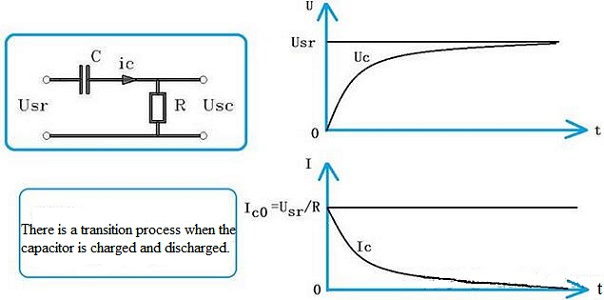Figure 1. Transition Process When Charging the Capacitor

The voltage across the capacitor is not allowed to change suddenly. So when the power is turned on, the voltage across the capacitor is equal to zero, and then the voltage rises exponentially until it enters a steady state. The capacitor after entering the steady state is equivalent to an open circuit. In fact, the capacitor can block the constant direct current and disconnect when it fully charged in the circuit. According to the leakage resistance of the capacitor, the charge can be stored in the capacitor for a long period of time.
When Usr is instantly added to the resistor-capacitor circuit, because the voltage across the capacitor is not allowed to change suddenly, the capacitor is equivalent to being short-circuited at this time. So at time 0, the current flowing through the capacitor and resistor R is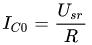.
Then the capacitor began the charging process, and the current became smaller and smaller. After 5 times the RC time, the capacitor charging is basically over and the current is reduced to zero. Since then, it has entered a steady state. The RC(τ) here is called the time constant.
We know that resistance is equal to the ratio of voltage to current, that is, R=U/I. We also know that the capacitance C is equal to the ratio of the electric quantity Q to the voltage U, and the electric quantity Q is equal to the product of the current I and the time t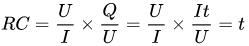.
It turns out that the product of resistance and capacitance is time. The unit of resistance is ohms and the unit of capacitance is farads, so the unit of time is seconds.
In Figure 1, when the capacitor is charged, the voltage across it is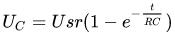.
We find Uc when t=0, 1RC, 2RC, 3RC, 4RC, and 5RC, as follows: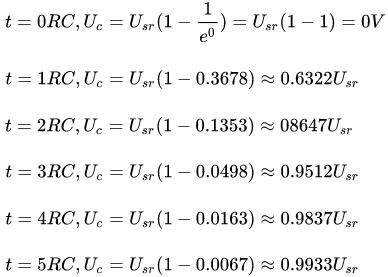It can be seen that when time t=0, the voltage across the capacitor is equal to zero; when t=5RC, the voltage across the capacitor is almost equal to the input voltage.
Let's look at the current flowing through the capacitor, its expression is as follows: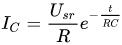When t=5RC, where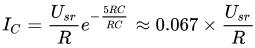.
It can be seen that the current at this time is almost equal to zero. Therefore, the transient process and steady-state process of the capacitor must be clearly distinguished.

## Ⅲ Capacitor Transient and Steady-state Processes

1) There are transient and steady-state processes in the capacitor charging circuit.
2) At the beginning of capacitor charging, it must be considered that the voltage across the capacitor does not allow sudden changes, which is an important principle.
3) The transient process generally ends after 5τ.
4) For Figure 1, at the moment of the transient start, the capacitor voltage Uc is equal to zero, and the current Ic is equal to the maximum value. We know from Ohm's law that the equivalent resistance of the capacitor is equal to zero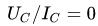. Usually we say that the capacitance at this time is equal to the short circuit i. In the steady state at the end of the transient, the capacitor voltage Uc is equal to the input voltage Usr, and the capacitor current Ic=0. According to the Ohm's law that the equivalent resistance of the capacitor is equal to infinity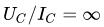. At this time, the capacitance is equivalent to an open circuit.
5) If the input signal voltage is a short pulse, the capacitor can transmit the signal to the load; if the input signal is a constant voltage, the capacitor will only respond during a short transition, and then block the input signal; if the input signal is an AC signal, which is exactly in the middle of the above two situations.
The higher the frequency of the AC signal, the easier it is to pass through the capacitor. We call this feature a high-pass filter function. Although the AC signal can pass through the capacitor, there will be a certain amount of clipping. This shows that the capacitor has the function of isolating DC in the steady state, and a high-pass characteristic. So we can further analyze, any circuit with capacitor and inductor, we must analyze the circuit according to the transient state and the steady state, in order to get the correct analysis result.

## Ⅳ Capacitor Circuit Analysis and Calculations

The analysis is available from the figure below: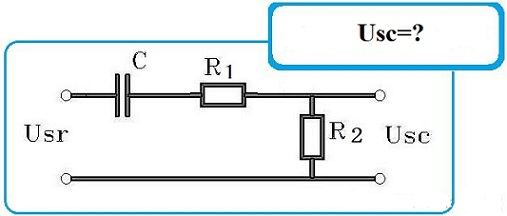Figure 2. Output Voltage Usc

If set Usr=10Vdc, the capacitance is equal to 10 microfarads, and the resistances R1 and R2 are both 1 kiloohm, then how to analyze the value of Usc?
Step 1: Determine the time constant of the capacitor.Figure 3. Usr in Short-circuit Connection

From the analysis of the above figure, it can be seen that the time constant is 20 milliseconds, and the time for 5 times the time constant is 0.1 seconds.
Step 2: Let's calculate the specific value of Usc.
When Usr in Figure is just established, the capacitor voltage drop is equal to zero, so there is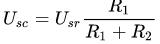.
After 5τ, the capacitor is full of voltage, and its value is Uc=Usr, so Usc=0,.
When charging starts, t=0,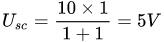When the time has passed 0.1 second, we have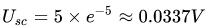, and the Usc at this time is almost equal to zero.

Now, let's connect R1 and C in parallel, and see what happens: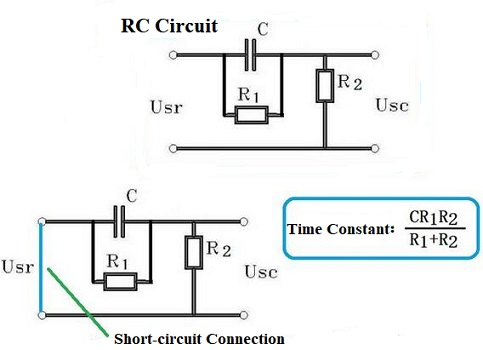Figure 4. R1 and C are Connected in Parallel

We see that if Usr in the figure is short-circuited, R1 and R2 are connected in parallel, so the time constant is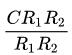At the beginning of Usr power on, C is equivalent to a short circuit, and Usr is directly loaded on both ends of the resistor R2, so at this time Usc=Use
When the circuit enters a steady state, Usc is equivalent to the partial pressure of Usr by resistors R2 and R1, namely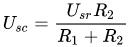Based on this, we can derive the following equation: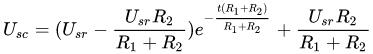.
In the above formula, the first term on the right side of the equal sign is the change in capacitor voltage, which reflects the transition process. The second term on the right side of the equal sign is the final steady-state voltage.
Substitute the parameters, and calculate the time constant first: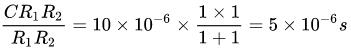.
In other words, when the time is 5τ, that is, 25 microseconds, the output voltage tends to stabilize. The final value is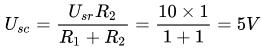.
It is still 5V, but the transition process is only 25 microseconds, which is much shorter than the previous 0.1 second.

## Ⅴ FAQ

1. When a capacitor is charging in a DC circuit?
At this point, the electric field between the plates cancels the effect of the electric field generated by the battery, and there is no further movement of charge. Thus, if a capacitor is placed in a DC circuit then, as soon as its plates have charged up, the capacitor effectively behaves like a break in the circuit.

2. What happens to the current in a DC circuit once a capacitor is charged?
For a capacitor charge Q = capacitance C multiplied by voltage V. This quite simply means that a rate of change of voltage gives rise to a current. If the voltage is rising linearly with time, the capacitor will take a constant current and once the voltage stops changing the current is zero.

3. Does current flow in a DC circuit while a capacitor is charging?
Yes. For DC circuits, when a capacitor is charged or discharged, current is flowing into and out of it. For AC circuits, a capacitor can act almost like a "resistor" but instead it is called reactance. But alas, current does flow through the capacitor.

4. Do capacitors charge with AC or DC?
When DC current is applied to a circuit with only resistance and capacitance, the capacitor will charge to the level of the applied voltage. Since DC only flows in one direction, once the capacitor is fully charged there is no more current flow.

5. Can we use capacitor in DC?
Capacitors can be used in many different applications and circuits such as blocking DC current while passing audio signals, pulses, or alternating current, or other time varying wave forms. ... At DC a capacitor has infinite impedance (open -circuit), at very high frequencies a capacitor has zero impedance (short-circuit).

6. Can a capacitor be charged by DC?
When capacitor is connected to dc voltage source, capacitor starts the process of acquiring a charge. This will built up voltage across capacitor. Once capacitor has acquire enough charge, current starts flowing and soon capacitor voltage reaches at value approximately equal to dc source voltage.

7. Why does AC pass through capacitor but not DC?
Capacitors have two parallel metallic plates placed close to each other and there is a gap between plates. A capacitor blocks DC but it allows AC. ... Therefore the electrons flowing in one direction (i.e. DC) cannot pass through the capacitor. But the electrons from AC source seem to flow through C.

8. What happens when capacitor is connected to DC?
When capacitors are connected across a direct current DC supply voltage, their plates charge-up until the voltage value across the capacitor is equal to that of the externally applied voltage. ... Then the Capacitance in AC circuits varies with frequency as the capacitor is being constantly charged and discharged.

9. Why capacitor is used in DC circuit?
Capacitors are useful to reduce the voltage pulsation. When the high voltage is applied to the parallel circuit, the capacitor is charged, and on the other hand, it is discharged with the low voltage. While electricity flowing out is alternating current, most of electronic circuits work with direct current.

10. Why do capacitors block DC current?
We know that there is no frequency i.e. 0Hz frequency in DC supply. If we put frequency “f = 0″ in the inductive reactance (which is AC resistance in capacitive circuit) formula. If we put XC as infinity, the value of current would be zero. That is the exact reason why a capacitor block DC.

11. How is a capacitor charged in a DC circuit?
When used in a direct current or DC circuit, a capacitor charges up to its supply voltage but blocks the flow of current through it because the dielectric of a capacitor is non-conductive and basically an insulator. ... At this point the capacitor is said to be “fully charged” with electrons.

12. Which capacitor is used in DC circuit?
Decoupling capacitor is used, where we have to decouple the two electronics circuits. In other words, the noise generated by one circuit is grounded by decoupling capacitor and it does not affect the performance of other circuit.

13. Can you charge a capacitor with DC current?
A DC voltage source is used to charge a Capacitor. When the DC voltage source is outputting more than the DC voltage source can charge, the Capacitor will charge up. Capacitors will charge up to 9 volts if they are connected to a 9-volt battery.

14. What happens if DC is applied to capacitor?
When capacitors are connected across a direct current DC supply voltage, their plates charge-up until the voltage value across the capacitor is equal to that of the externally applied voltage. ... Then the Capacitance in AC circuits varies with frequency as the capacitor is being constantly charged and discharged.

15. Can we charge capacitor with DC current?
When capacitor is connected to dc voltage source, capacitor starts the process of acquiring a charge. This will built up voltage across capacitor. Once capacitor has acquire enough charge, current starts flowing and soon capacitor voltage reaches at value approximately equal to dc source voltage.